# Class 9 NCERT Solutions- Chapter 8 Quadrilaterals – Exercise 8.2

• Difficulty Level : Easy
• Last Updated : 13 Jan, 2021

### Question 1. ABCD is a quadrilateral in which P, Q, R, and S are mid-points of the sides AB, BC, CD, and DA (see Fig 8.29). AC is a diagonal. Show that:

(i) SR || AC and SR = ½ AC

(ii) PQ = SR

Hey! Looking for some great resources suitable for young ones? You've come to the right place. Check out our self-paced courses designed for students of grades I-XII

Start with topics like Python, HTML, ML, and learn to make some games and apps all with the help of our expertly designed content! So students worry no more, because GeeksforGeeks School is now here!

(iii) PQRS is a parallelogram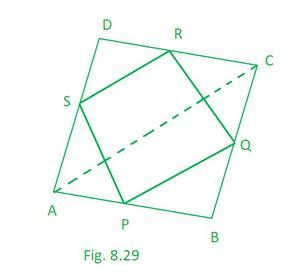Solution:

Given that, P, Q, R and S are the mid points of quadrilateral ABCD

Theorem 8.9: The line segment joining the mid-points of two sides of a triangle is parallel to the third side.

(i) So here, taking ∆ACD

we can see S and R are the mid points of side AD and DC respectively.    [Given]

Hence, SR || AC and SR = ½ AC (NCERT Theorem 8.9)……………………….(1)

(ii) So here, taking ∆ACB

we can see P and Q are the mid points of side AB and BC respectively.    [Given]

Hence, PQ || AC and PQ = ½ AC (NCERT Theorem 8.9)…………………………(2)

From (1) and (2) we can say,

PQ = SR

(iii) so from (i) and (ii) we can say that

PQ || AC and SR || AC

so, PQ || SR and PQ = SR

If each pair of opposite sides of a quadrilateral is equal, then it is a parallelogram.

Hence, PQRS is a parallelogram.

### Question 2. ABCD is a rhombus and P, Q, R and S are the mid-points of the sides AB, BC, CD and DA respectively. Show that the quadrilateral PQRS is a rectangle.

Solution:

Given that, P, Q, R and S are the mid points of Rhombus ABCD.

Theorem 8.9: The line segment joining the mid-points of two sides of a triangle is parallel to the third side.

Construction: Join AC and BD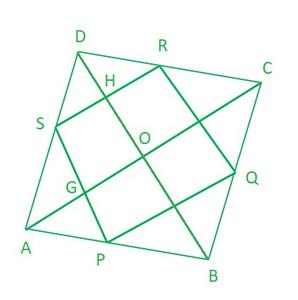So here, taking ∆ABD

We can see P and S are the mid points of side AB and AD respectively.    [Given]

Hence, PS || BD and PS = ½ BD (NCERT Theorem 8.9)……………………….(1)

Similarly, is we take ∆CBD

We can see R and Q are the mid points of side CD and CB respectively.    [Given]

Hence, RQ || BD and RQ = ½ BD (NCERT Theorem 8.9)……………………….(2)

So from (1) and (2), we conclude that

PS || RQ and PS = RQ

If each pair of opposite sides of a quadrilateral is equal, then it is a parallelogram.

Hence, PQRS is a parallelogram.

Now in ∆ACD

We can see S and R are the mid points of side AD and CD respectively.    [Given]

Hence, SR || AC and RS = ½ AC (NCERT Theorem 8.9)

from (2) RQ || BD and RQ = ½ BD (NCERT Theorem 8.9)

Hence, OGSH is a parallelogram.

∠HOG = 90° (Diagonal of rhombus intersect at 90°)

So ∠HSG = 90° (opposite angle of a parallelogram are equal)

As, PQRS is a parallelogram having vertices angles equal to 90°.

Hence, PQRS is a Rectangle.

### Question 3. ABCD is a rectangle and P, Q, R, and S are mid-points of the sides AB, BC, CD, and DA respectively. Show that the quadrilateral PQRS is a rhombus.

Solution:

Given that, P, Q, R and S are the mid points of Rectangle ABCD.

Construction: Join AC

Theorem 8.9 : The line segment joining the mid-points of two sides of a triangle is parallel to the third side.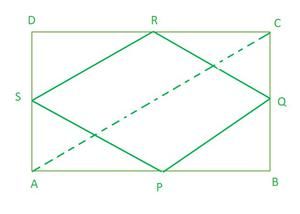So here, taking ∆ACD

we can see S and R are the mid points of side AD and DC respectively.    [Given]

Hence, SR || AC and SR = ½ AC (NCERT Theorem 8.9)……………………….(1)

Now, taking ∆ACB

we can see P and Q are the mid points of side AB and BC respectively.    [Given]

Hence, PQ || AC and PQ = ½ AC (NCERT Theorem 8.9)…………………………(2)

So from (1) and (2), we conclude that

SR || PQ and SR = PQ

Hence, PQRS is a parallelogram. (If each pair of opposite sides of a quadrilateral is equal, then it is a parallelogram)

Now in ∆QBP and ∆QCR

QC = QB (Q is the mid point of BC)

RC = PB (opposite sides are equal, hence half length is also equal)

∠QCR = ∠QBP (Each 90°)

∆QBP ≅ ∆QCR (By SAS congruency)

QR = QP (By C.P.C.T.)

As PQRS is a parallelogram and having adjacent sides equal

Hence, PQRS is a rhombus

### Question 4. ABCD is a trapezium in which AB || DC, BD is a diagonal and E is the mid-point of AD. A line is drawn through E parallel to AB intersecting BC at F (see Fig. 8.30). Show that F is the mid-point of BC.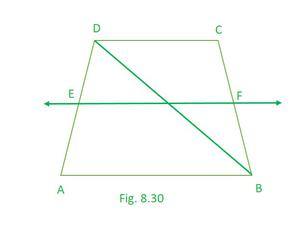Solution:

Let O be the point of intersection of lines BD and EF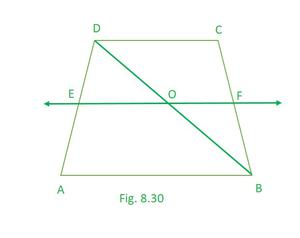Theorem 8.10 : The line drawn through the mid-point of one side of a triangle, parallel to another side bisects the third side.

we can see S is the mid point of side AD and ED || AB   [Given]

Hence, OD = ½ BD ………..(NCERT Theorem 8.10)

Now, taking ∆BCD

we can see O is the mid point of side BD and OF || AB   [Proved and Given]

Hence, CF = ½ BC…….. (NCERT Theorem 8.10)

Hence proved!!

### Question 5. In a parallelogram ABCD, E and F are the mid-points of sides AB and CD respectively (see Fig. 8.31). Show that the line segments AF and EC trisect the diagonal BD.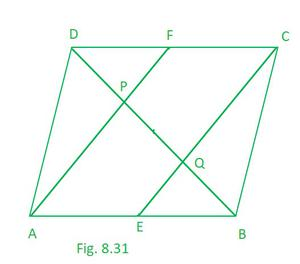Solution:

Theorem 8.10 : The line drawn through the mid-point of one side of a triangle, parallel to another side bisects the third side.

Given, E and F are the mid points of side AB and CD of parallelogram ABCD.

As, AB || CD and AB = CD  (opposite sides of parallelogram)………..(1)

AE = CF  (halves of opposite sides of parallelogram)………………………(2)

from (1) and (2)

AECF is a parallelogram

Hence, AF || EC

Now taking ∆APB

we can see E is the mid point of side AB and EF || AP   [Given and proved]

Hence, BQ = PQ………..(NCERT Theorem 8.10)………………….(1)

Now taking ∆CQD

we can see F is the mid point of side CD and CQ || FP   [Given and proved]

Hene, DP = PQ………..(NCERT Theorem 8.10)………………..(2)

From (1) and (2) we conclude that,

BQ = PQ = DQ

Hence, we can say that line segments AF and EC trisect the diagonal BD

### Question 6. Show that the line segments joining the mid-points of the opposite sides of a quadrilateral bisect each other.

Solution:

Theorem 8.9 : The line segment joining the mid-points of two sides of a triangle is parallel to the third side.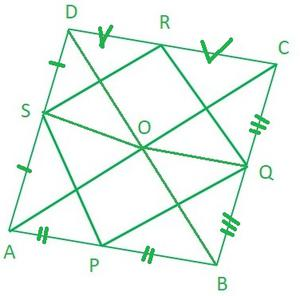So here, taking ∆ACD

we can see S and R are the mid points of side AD and DC respectively.    [Given]

Hence, SR || AC and SR = ½ AC (NCERT Theorem 8.9)……………………….(1)

Now, taking ∆ACB

we can see P and Q are the mid points of side AB and BC respectively.    [Given]

Hence, PQ || AC and PQ = ½ AC (NCERT Theorem 8.9)…………………………(2)

So from (1) and (2), we conclude that

SR || PQ and SR = PQ

Hence, PQRS is a parallelogram. (If each pair of opposite sides of a quadrilateral is equal, then it is a parallelogram)

And since the diagonal of parallelogram bisects each other

so, QS and PR bisects each other.

### Question 7. ABC is a triangle right angled at C. A line through the mid-point M of hypotenuse AB and parallel to BC intersects AC at D. Show that

(i) D is the mid-point of AC

(ii) MD ⊥ AC

(iii) CM = MA = ½ AB

Solution:

Theorem 8.10 : The line drawn through the mid-point of one side of a triangle, parallel to another side bisects the third side.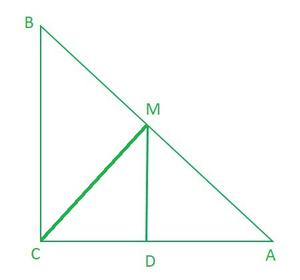(i) while taking ∆ABC

we can see M is the mid point of side AB and DM || BC   [Given]

This implies, DC= AD ……….. (NCERT Theorem 8.10)

Hence, D is the mid-point of AC.

(ii) As we know MD || BC and AC is transversal

This implies, ∠ACB = ∠ADM = 90°

Hence, MD ⊥AC

AD = CD (D is the mid point of AC (Proved))

∠CDM = ∠ADM (proved, MD ⊥AC)

DM = DM (common)

∆ADM ≅ ∆CDM (By SAS congruency)

CM = AM (By C.P.C.T.)

CM = AM = ½ AB (M is the mid point of AB)

My Personal Notes arrow_drop_up